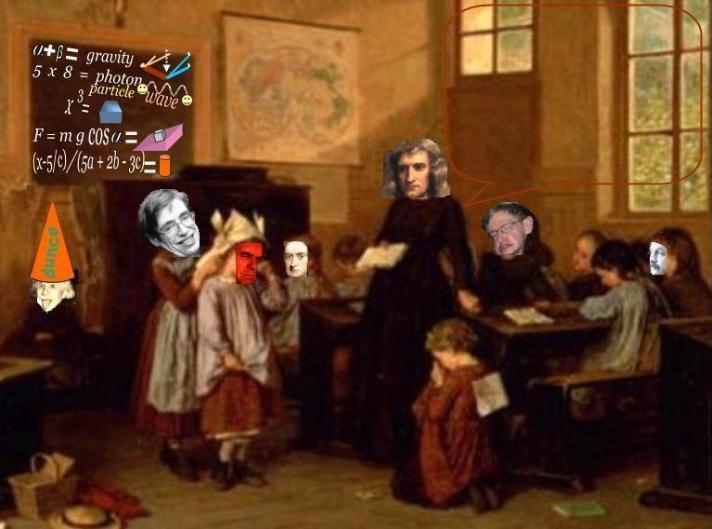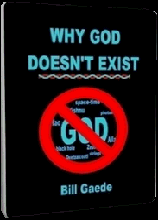Summary

Contemporary Mathematical Physics has three pillars: General Relativity (GR), Quantum Mechanics (QM),
and String Theory (ST).  All three of these fields of study have their foundations in Geometry. GR alleges
that gravity is a result of the geometry of space, QM relies on geometric figure known as a point, and ST
invokes the straight line. Without Geometry, these branches of modern theoretics collapse.

Unfortunately, the mathematicians have misconstrued the figures, axioms, and definitions of Geometry,
and use them inconsistently anyways. This is not a case where we merely need to tweak the process a
little bit or erase the board completely and start over. It is rather an impossible task. Geometry is a
surrealistic discipline that purports to describe the static world of Physics with the dynamic language of
Mathematics. The mathematicians have confused points with locations, distance with displacement,
dimensions and coordinates with number lines, and solids with volumes. After 3000 years of history and
experience, the mathematicians can't even tell you what a number is.

Mathematics is a science of behavior. Physics is the science of existence. Mathematics only studies
adverbs (relations, how something moves, etc.). Physics deals with the remaining grammatical categories.
Mathematical Physics deals with what an observer measures and perceives. Physics is observer-free.
Physics deals with 'what is' irrespective of testimony or artificial standards. Mathematics is not only NOT
the language of Physics, it has absolutely nothing to do with Physics.  In Einstein's words:

" Insofar as mathematics is true, it does not describe the real world. Insofar as it
describes the real world, it is not true."

" Physics is built on the basic and rather wistful hypothesis that Mother Nature
doesn't know much math. Remainders and constants are men's crumbs, not
hers" 

Math is Math and Physics is Physics. Never the twain shall meet. The attempt to reconcile them results in
a religion known as Mathematical Physics.

Module main page: Main Page

Pages in this module:

 Mathematics isNOTthe language of PhysicsHow can you expect to understand anything about the Universe if you don't study Math, Bill?
 Adapted for the Internet from:Why God Doesn't Exist________________________________________________________________________________________